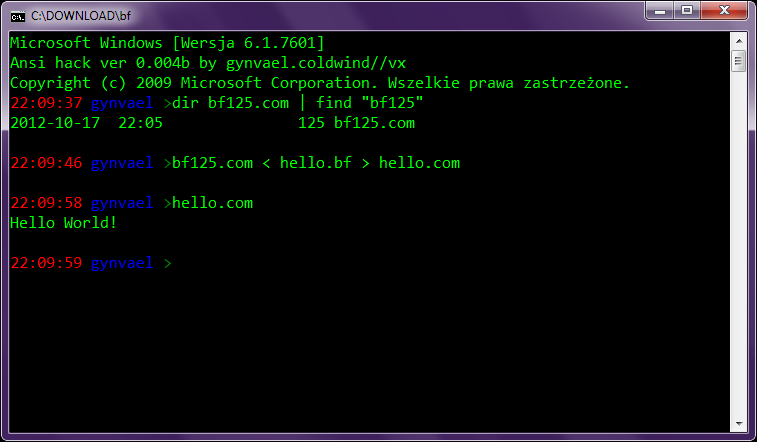Brainfuck compiler in 125 bytes (last update: 2013-01-09, created: 2012-10-17) back to the list ↑
A long time ago (in 2006) on a random IRC channel a friend of mine (fr3m3n, Hi!) came up with an idea, to make a compo (competition) for "the smallest Brainfuck compiler". The rules were:
• The BF input source code was to be read from stdin.
• The output executable was to be a DOS com file.
• The output was to be written out to stdout.
• The compiler had to properly compile three test files, out of which the last one was a bf hello world:
`++++++++++[>+++++++>++++++++++>+++>+<<<<-]>++.>+.+++++++..+++.>++.<<+++++++++++++++.>.+++.------.--------.>+.>.`
• The compiler with the smallest compiler executable file wins! (the size of compiler-source-bf file and the size of the source code of the compiler did not matter).
• (I don't remember now, but there were some rules about what can be assumed about the initial registry values)
I don't have to add that we all went for creating .com-compilers as .com files, right? :)

The contestants were j00ru, adam_i, fr3m3n and yours truly, and the results were (not sure if these were the final ones - these are the ones I found on my HDD):
• j00ru, 163 bytes
• me, 125 bytes
• fr3m3n, 140 bytes (+-3 bytes, I used a different compiler now to compile his code)
However the order of the results is of no importance here, since all of the codes were quite impressive and used a lot of hacky assembly code.

That said, I did find my source code on the disk (actually I found the sources for all the entries, but I need to ping the authors whether I can publish them), so I decided to post it here (scroll down if you're interested).

A couple of notes about this though. But first, a proof it works on Windows 7 NTVDM:Sadly, that's not the case for DOSBox - it generates some faulty code and hangs. I did not dig into the details, but probably it has something to do with the assumptions about the initial values of the registers or flags.

Furthermore, the compiler works OK with the hello world code from the rules, and with a few more simple programs. That said, it doesn't generate proper code for more complicated code. Again, I did not dig into this, so I don't know why. By design it should be a proper compiler, but I guess something went astray during the executable-size-optimization process.

Still, I decided to post this as a note, since someone might find some ideas I used in the code entertaining. You can also compare differences between versions to see how exactly the size optimization was achieved (see link below).

(random note to self: we must repeat a similar compo some day; this one was fun :)

UPDATE: Peter Ferrie made a 109 byte version. This is getting serious ;>

### Appendix: Gynvael's bf125.com

Note: Other versions (source + binaries) and the test file are available here.

```[bits 16] [org 0x100] ; assume bp=091e used ; assume di=fffe ; assume si=0100 ; assume dx=???? ; assume cx=00ff used ; assume bx=0000 used ; assume ax=0000 used ; assume sp=fffe ; ------------------------------------- start:   ;mov al, code_start_end - start   mov al, code_nothing - start code_start:   mov ch, 0x75   push cx   mov di, cx   rep stosb   pop di   jmp short code_start_end ; ------------------------------------- find_right:   pop bx   ;assume cx = 0   xor [di], cl ; prev: test byte [di], 0xff   jnz short loop_right_end_2   inc cx        loop_right:     inc bx     mov al, [bx]     cmp al, 0xC3 ; ret     jne short loop_right_sth     dec cx     jz short loop_right_end     loop_right_sth:     cmp al, 0xBB ; mov bx     jne short loop_right     inc cx     jmp short loop_right        loop_right_end_2:   ; stupid nasm can't do good lea   ; lea ax, [bx - 5]   db 0x8d, 0x47, - (code_sqleft_next_end - code_sqleft_next)   push ax    loop_right_end:   inc bx   push bx    code_sqright:   code_sqright_next:   ret   code_sqright_next_end:   code_start_end:   db '\$'   db 0xff ; ------------------------------------- real_start:   ; create lookup table   ; mov byte [di+'+'], code_inc - start   ; stupid nasm can't make mov byte [di+byte '+'] --;   ;db 0xC6, 0x45, '<', code_left - start   inc byte [di+'<']   ;db 0xC6, 0x45, '>', code_right - start   dec byte [di+'>']   db 0xC6, 0x45, '[', code_sqleft - start   db 0xC6, 0x45, ']', code_sqright - start   lea sp, [di+45+2]   push (code_dec - start) + (code_dot - start) * 256   push (code_inc - start) + (code_comma - start) * 256   ; assume sp 43   ; startup code   mov dx, code_start   jmp short write pre_write:   ; switch   xchg al, bl   mov dl, [di+bx]    write:   ; write   mov ax, bp   ; mov ah, 9   int 0x21      ; read   mov ah, 0x06   mov dl, 0xff   int 0x21   jnz pre_write    ;----------------------------------------------- the_end:   mov dl, code_end - start   ;mov dx, code_end   ;mov ah, 9   mov ax, bp   int 0x21 code_end:   mov ah, 0x4c   int 0x21 code_inc:    inc byte [di]    db '\$' code_sqleft:   code_sqleft_next:   mov ax, 0x100 + find_right - code_start   call ax   code_sqleft_next_end: code_right:    inc di code_nothing   db '\$' code_left:    dec di   db '\$'    code_dec:    dec byte [di]    db '\$' code_comma:   mov byte [di], 0xff code_dot:    mov dl, [di]   mov ah, 6   int 0x21   mov [di], al   db '\$' ```
【 design & art by Xa / Gynvael Coldwind 】 【 logo font (birdman regular) by utopiafonts / Dale Harris 】